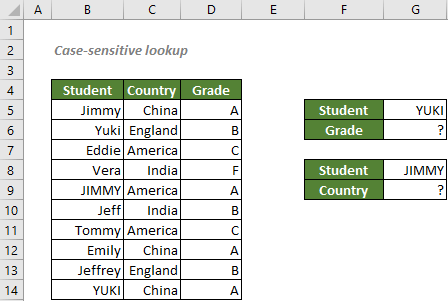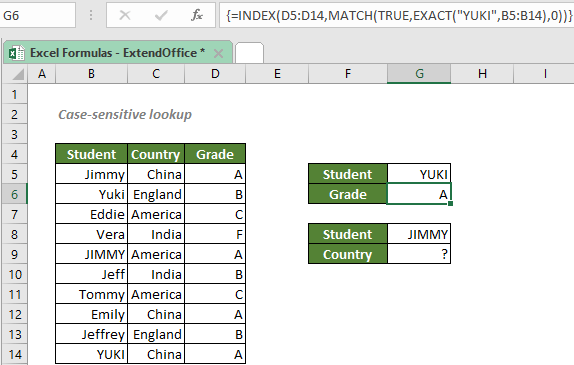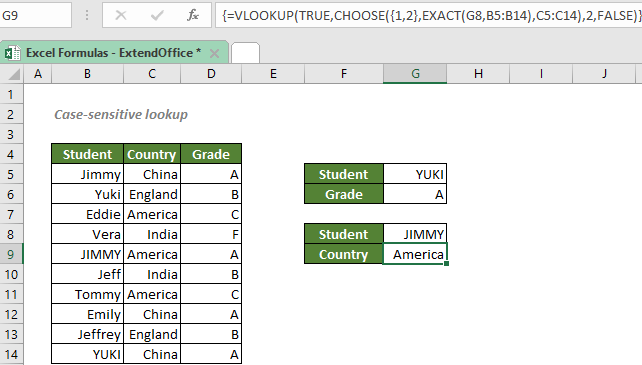## Case-sensitive lookup

You may know that you can combine the INDEX and MATCH functions, or use the VLOOKUP function to lookup values in Excel. However, the lookups aren’t case-sensitive. So, in order to perform a case-sensitive match, you should take advantage of the EXACT and unctions.#### Perform a case-sensitive lookup with INDEX and MATCH

To know the grade of YUKI as shown in the screenshot above with INDEX and MATCH, you can use the EXACT function to compare the text strings in the students’ name range to YUKI, the value in the cell G5, including the case of each character. Then we can use the INDEX and MATCH functions together to find the value we want.

#### Generic syntax

=INDEX(return_range,MATCH(TRUE,EXACT(lookup_value,lookup_range),0))

√ Note: This is an array formula that requires you to enter with Ctrl + Shift + Enter.

• return_range: The range where you want the combination formula to return the value from. Here refers to the grade range.
• lookup_value: The value EXACT used to perform a case-sensitive comparison with the text strings in the lookup_range. Here refers to the given name, YUKI.
• lookup_range: The range of cells to compare with the lookup_value. Here refers to the name range.
• match_type 0: MATCH will find the first value that exactly equals to the lookup_value.

To know the grade of YUKI, please copy or enter the formula below in the cell G6, and press Ctrl + Shift + Enter to get the result:

=INDEX(D5:D14,MATCH(TRUE,EXACT("YUKI",B5:B14),0))

Or, use a cell reference to make the formula dynamic:

=INDEX(D5:D14,MATCH(TRUE,EXACT(G5,B5:B14),0))#### Explanation of the formula

=INDEX(D5:D14,MATCH(TRUE,EXACT("YUKI",B5:B14),0))

• EXACT(G5,B5:B14): The EXACT function compares the text strings in the students’ name range B5:B14 against "YUKI", the value in the cell G5, returns a TRUE if a value in the cells through B5 to B14 is exactly the same as YUKI, a FALSE otherwise. So, we will get an array of TRUEs and FALSEs like this:
{FALSE;FALSE;FALSE;FALSE;FALSE;FALSE;FALSE;FALSE;FALSE;TRUE}
• MATCH(TRUE,EXACT(G5,B5:B14),0) = MATCH(TRUE,{FALSE;FALSE;FALSE;FALSE;FALSE;FALSE;FALSE;FALSE;FALSE;TRUE},0): The match_type 0 asks the MATCH function to find the exact match. The function will then return the position of its exact lookup_value “TURE” in the array, which is 10, since the only TRUE is at the 10th position in the array.
• INDEX(D5:D14,MATCH(TRUE,EXACT(G5,B5:B14),0)) = INDEX(D5:D14,10): The INDEX function returns the 10th value in the grade range D5:D14, which is A.

#### Perform a case-sensitive lookup with VLOOKUP

To know the country that JIMMY comes from with the VLOOKUP function, you should take advantage of the CHOOSE and EXACT functions to perform a case-sensitive lookup. The EXACT function will conduct a case-sensitive comparison between JIMMY and each text string in the students’ name range. Then we can use CHOOSE to combine the EXACT’s result and the values in the country range. Now it’s time for VLOOKUP to show its ability.

#### Generic syntax

=VLOOKUP(TRUE,CHOOSE({1,2},EXACT(lookup_value,lookup_range),return_range),2,FALSE)

√ Note: This is an array formula that requires you to enter with Ctrl + Shift + Enter.

• lookup_value: The value EXACT used to perform a case-sensitive comparison with the text strings in the lookup_range. Here refers to the given name, JIMMY.
• lookup_range: The range of cells to compare with the lookup_value. Here refers to the name range.
• return_range: The range where you want the combination formula to return the value from. Here refers to the country range.
• range_lookup FALSE: The VLOOKUP function will search for the exact match.

To know the country JIMMY comes from, please copy or enter the formula below in the cell G9, and press Ctrl + Shift + Enter to get the result:

=VLOOKUP(TRUE,CHOOSE({1,2},EXACT("JIMMY",B5:B14),C5:C14),2,FALSE)

Or, use a cell reference to make the formula dynamic:

=VLOOKUP(TRUE,CHOOSE({1,2},EXACT(G8,B5:B14),C5:C14),2,FALSE)#### Explanation of the formula

=VLOOKUP(TRUE,CHOOSE({1,2},EXACT(G8,B5:B14),C5:C14),2,FALSE)

• EXACT(G8,B5:B14): The EXACT function compares the text strings in the students’ name range B5:B14 against the value in the cell G8, JIMMY, returns a TRUE if a value in the students’ name range is exactly the same as JIMMY, a FALSE otherwise. So, we will get an array of TRUEs and FALSEs like this:
{FALSE;FALSE;FALSE;FALSE;TRUE;FALSE;FALSE;FALSE;FALSE;FALSE}
• CHOOSE({1,2},EXACT(G8,B5:B14),C5:C14) = CHOOSE({1,2},{FALSE;FALSE;FALSE;FALSE;TRUE;FALSE;FALSE;FALSE;FALSE;FALSE},C5:C14): The index_num argument of CHOOSE {1,2} combines the EXACT array and the values from C5:C14 into a two-dimensional array like this:
{FALSE,"China";FALSE,"England";FALSE,"America";FALSE,"India";TRUE,"America";FALSE,"India";FALSE,"America";FALSE,"China";FALSE,"England";FALSE,"China"}
• VLOOKUP(TRUE,CHOOSE({1,2},EXACT(G8,B5:B14),C5:C14),2,FALSE) = VLOOKUP(TRUE,{FALSE,"China";FALSE,"England";FALSE,"America";FALSE,"India";TRUE,"America";FALSE,"India";FALSE,"America";FALSE,"China";FALSE,"England";FALSE,"China"},2,FALSE): The range_lookup FALSE askes the VLOOKUP function to search for the exact value “TRUE” in the 1st column of the two-dimensional array, and returns its exact match in the 2nd column, which is America.

#### Related functions

Excel INDEX function

The Excel INDEX function returns the displayed value based on a given position from a range or an array.

Excel MATCH function

The Excel MATCH function searches for a specific value in a range of cells, and returns the relative position of the value.

Excel VLOOKUP function

The Excel VLOOKUP function searches for a value by matching on the first column of a table and returns the corresponding value from a certain column in the same row.

Excel EXACT function

The EXACT function compares two strings and returns TRUE if they are exactly same (taking into account case sensitivity), or returns FALSE.

Excel CHOOSE function

The CHOOSE function returns a value from the list of value argument based on the given index number. For example, CHOOSE(3,”Apple”,”Peach”,”Orange”) returns Orange, the index number is 3, and Orange is the third value after index number in the function.

#### Related Formulas

Exact Match With INDEX And MATCH

If you need to find out the information listed in Excel about a specific product, movie or a person, etc., you should make a good use of the combination of INDEX and MATCH functions.

Count cells that contain specific text with case sensitive

In this tutorial, you will learn how to use a formula with SUMPRODUCT, ISNUMBER and FIND functions to count cells that contain specific text, taking into account upper and lower case.

### The Best Office Productivity Tools

#### Kutools for Excel - Helps You To Stand Out From Crowd

 Popular Features: Find, Highlight or Identify Duplicates  |  Delete Blank Rows  |  Combine Columns or Cells without Losing Data  |  Round without Formula ... Super VLookup: Multiple Criteria  |  Multiple Value  |  Across Multi-Sheets  |  Fuzzy Lookup... Adv. Drop-down List: Easy Drop Down List  |  Dependent Drop Down List  |  Multi-select Drop Down List... Column Manager: Add a Specific Number of Columns  |  Move Columns  |  Toggle Visibility Status of Hidden Columns  |  Compare Columns to Select Same & Different Cells ... Featured Features: Grid Focus  |  Design View  |  Big Formula Bar  |  Workbook & Sheet Manager | Resource Library (Auto Text)  |  Date Picker  |  Combine Worksheets  |  Encrypt/Decrypt Cells  |  Send Emails by List  |  Super Filter  |  Special Filter (filter bold/italic/strikethrough...) ... Top 15 Toolsets:  12 Text Tools (Add Text, Remove Characters ...)  |  50+ Chart Types (Gantt Chart ...)  |  40+ Practical Formulas (Calculate age based on birthday ...)  |  19 Insertion Tools (Insert QR Code, Insert Picture from Path ...)  |  12 Conversion Tools (Numbers to Words, Currency Conversion ...)  |  7 Merge & Split Tools (Advanced Combine Rows, Split Excel Cells ...)  |  ... and more

Kutools for Excel Boasts Over 300 Features, Ensuring That What You Need is Just A Click Away...#### Office Tab - Enable Tabbed Reading and Editing in Microsoft Office (include Excel)

• One second to switch between dozens of open documents!
• Reduce hundreds of mouse clicks for you every day, say goodbye to mouse hand.
• Increases your productivity by 50% when viewing and editing multiple documents.
• Brings Efficient Tabs to Office (include Excel), Just Like Chrome, Edge and Firefox.